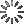• Share

# HTML5 Canvas Particles Web MatrixKushagra Agarwal

This is another experiment that I recently did with the awesome `canvas` element which I am in love with. The main concept here is that there are some particles on the canvas which start attracting other when they come close to each other. Also, they'll start drawing lines between them as they come closer to each other and the opacity of the lines depends upon the distance between them. If the distance is larger, then the opacity will be lower. Also, there is a minimum distance and after covering that distance only the particles will start to attract each other.

I've written many comments in the JS code to make sure that you understand everything perfectly. Even after looking at the code you don't understand something. then ask in the comments and I'll help you as soon as possible.

Here's some basic explanation of some of the most important logic and concepts. First of all, take a look at how I created the web of particles.

```ctx.beginPath();
ctx.strokeStyle = "rgba(255,255,255,"+ (1.2-dist/minDist) +")";
ctx.moveTo(p1.x, p2.y);
ctx.lineTo(p2.x, p2.y);
ctx.stroke();
ctx.closePath();
```

This is the basic thing that is used to draw the lines from one particle's center point to another particle's center point when the distance between them is larger than the `minDist` variable. Also, the opacity of the line is varying inversely with the distance between the particles. This gives a very cool effect.

Now about the acceleration, I just made the velocity vary linearly with the distance between the particles so they get accelerated according to how much closer they are. Here's the code for it.

```// Some acceleration for the partcles
// depending upon their distance
var ax = dx/2000,
ay = dy/2000;

// Apply the acceleration on the particles
p1.vx -= ax;
p1.vy -= ay;

p2.vx += ax;
p2.vy += ay;
```

Other things like movement of particles are very basic and if you don't understand that, then take a look at some of my previous tutorials and examples based on this part only. Have a nice day :)

### Validations

(Errors from your JS or Pre-Processors code)

HTML
CSS
JavaScript

CSSDeck G+